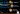Home>Data Engineering>AI/ML>Can a specific algorithm solve Machine Learning problems?

# Can a specific algorithm solve Machine Learning problems?

Trying to find the best algorithm suitable for the machine learning project is trial and error. Of course, hit and trial always work but understanding the process can save us time. Understanding the types of machine learning and its algorithms will help choose an optimized algorithm.

Supervised machine learning

The machine learns under guidance using labeled datasets to train algorithms that classify data or predict outcomes accurately. Training data given to the machine will work as a teacher and teaches the machine to predict data accurately.

Unsupervised machine learning

To act without any supervision or anybody’s direction. It’s a learning technique in which models are not supervised using a training dataset. Instead, models itself. The machine has to figure out the dataset given and find the hidden patterns to make predictions about the output accordingly.

Reinforcement machine learning

To establish or encourage a behavior. Machine learning training method based on rewarding desired behaviors and/or punishing undesired ones. It follows the hit and trial method and learns from experience.## Algorithms and their concepts for Supervised Machine Learning

Linear regression algorithms are all about finding the best fit line through data. The regression line is the best fit line. Logistic regression is the adaptation of linear regression and predicts the probability of occurrence of an event by fitting data to a logit function. Both algorithms are easy to understand and fast to train. These are the types of machine learning algorithms that are used for small data classification problems. Accuracy is a major concern where these algorithms lack, and the model occasionally ends up overfitting.

Second comes the Nearest Neighbours, which helps in the recommended products section. It finds sample data closest to the distance in the target object. For Example- the product recommended section you see on eCommerce. It is based on the data of previous maximum product searches by users. This algorithm is very accurate, simply adaptable, and very easy to understand. However, it is not commonly used because of the cost and slow performance due to IO operations, and choosing the wrong distance measure can lead to inaccurate results.

Forecasting data which is based on multiple decisions with the help of large data sets, is done by Random Forest. Provides high accuracy with fast results, but this is not suitable for small samples and is very slow at training.

## K-Means Most Suitable for Unsupervised Learning?

Trying to find a pattern in the data is what unsupervised learning does. K stands for the number of clusters in an algorithm. Finding nearest clusters for each data point so that the sum of squared distance between clusters and data point should be minimum. It is swift, and clusters can be calculated using K-means. It works flawlessly when the sizes of clusters are the same. Some factors might be problematic, like each data point must have numerical attributes as it won’t with categorical values. It won’t work well if the clusters overlap or the data is irregular.

Keeping it simple always works on many problems.

If you are getting desired results from simple algorithms, you don’t need to set parameters. According to research, most people use simple algorithms like regression because they solve all everyday business problems with simple algorithms like XG Boost.

## Reinforcement Learning

Behavioral response from a machine by taking actions where positive feedback gets a reward and negative feedback get a penalty. Most commonly used algorithms in reinforcement learning in Q-learning – another types of machine learning.

Q-learning works on estimating an action for the machine. The machine performs an action and gets a reward. Then again, it serves the following action with the highest value, and from the result, it updates the previous action.

Supervised learning is widely used in forecasting risks and predicting sales, and so on. For Example, regression problems can be solved by linear or logistic regression, and classification problems can be solved by random forest.

We see the recommendations on eCommerce and Credit card fraud detection by unsupervised learning. K- Means is used for Cluster analysis, and a priori is used for association problems.

Reinforcement learning is mainly used in self-driving cars and gaming, where Q-leaning is the most used algorithm.